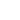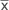Abstract Abstracts8.5 Statistics Parasitological data collected in the laboratory or in the field show all kinds of frequency distributions, the values of which can be obtained either as discrete measurements (such as the number of worms per individual host) or continuous measurements (such as the length of duration of patency). The results from complex chains of factors, often affecting only a few, but highly parasitized individuals, therefore usually do not follow the statistical laws of the normal and binomial distribution. Accordingly, the characteristics of the Poisson distribution (fig. 8.7, page 304) and the negative binomial distribution (fig. 8.8, page 305) are presented. The dispersion coefficient of discrete frequencies (variance s2 divided by the arithmetic mean) indicates the type of distribution: <1 positive binomial, >1 negative binomial, =1 Poisson distribution. The shape of these distributions becomes asymmetric when the arithmetic meanis low. Ranking tests are useful for comparing two sets of data and are so-called parameter-free. However, two conditions have to be fulfilled: only truly different values can be attributed to different ranks and the basic entities of the two sets of data must distribute steadily and in the same manner. In parasitological experiments these criteria are not always fulfilled. Sequence testing is useful for the analysis of ongoing clinical experiments, because a statistically significant difference between two treatment groups becomes immediately recognizable by graphical evaluation (fig. 8.9, page 306). The principles of the probability error and the null and the alternative hypothesis are explained. As an example of the way in which a parasite may distribute either in its vector or in its definitive host, the negative binomial distribution of third stage metacyclic larvae of a filarial parasite (Acanthocheilonema viteae in the vector tick) is given, together with the resulting total load of adult worms per rat after increasing the infestation doses given either as single or as multiple administrations (fig. 8.6, page 303).start